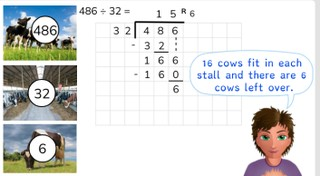Long division with a number to 1,000 with remainder

# Long division with a number to 1,000 with remainder

Students learn long division with a number to 1,000 with remainder.8,000 schools use Gynzy92,000 teachers use Gynzy1,600,000 students use Gynzy

## General

The students learn long division with a number to 1,000 with remainder.

## Standards

CCSS.Math.Content.6.NS.B.2

## Learning objective

Students will be able to use long division to divide a number to 1,000 by a number greater than 10 with remainder.

## Introduction

The students read the statement. If they think that it is true, they stand up. If they think that the statement is false, then they stay seated. Also ask students that think that the statement is incorrect, what they think a correct version of the statement would be.

## Instruction

Explain that with long division you put the numbers of the problem next to one another. First you write the divisor on the left. Then you write the dividend on the right and draw a box around it. You will write the answer on top of the box. Show that you first look at the first digit of the dividend. That is the 6 of 652. You cannot divide this 6 by 18, so you also need the next digit. Next you see how many times you can divide 65 by 18. Emphasize that to help, you can make a helping row of the table of 18. In this way you can quickly check what the largest number is that you can take away. In this case the largest number is: 3 × 18 = 54. Write the number 54 under the 65 and subtract them from each other. You write the answer (11) underneath. You write the 3, from 3 × 18, in the answer on top of the box. 18 does not fit into 11, so you look again at the next digit. Write the 2 of 652 next to the 11. Now you have 112. Now you look again to see what the largest number is that you can take away. That is 6 × 18 = 108. Write the 108 under the number 112 and subtract these numbers from each other. You now have 4 left over. You can no longer divide 4 by 18 so this is your remainder. You write the 6 of 6 × 18 next to the 3 in the answer. Your outcome is now 36 R 4. Explain that you will sometimes be asked for the remainder, but there is no remainder. In that case you write a 0 as the remainder. Next you can practice the problem 907 ÷ 20 = together with the students. You may remove the cover to show the helping row. Now have the students solve the next three problems on their own.

Check whether the students can use long division with a number to 1,000 with remainder, by asking the following question:
- What steps do you follow to solve a problem using long division?

## Quiz

The students test their understanding of long division with a number to 1,000 with remainder through ten exercises. The exercises are all division problems that should be solved using long division. In some of the problems the divisor is a one-digit number, and in other problems the divisor is a two-digit number.

## Closing

Discuss once again the importance of being able to use long division to divide a number to 1,000 with remainder. As a closing activity you have the students solve problems in pairs using long division. The students first solve a problem individually and then check the answer and steps together with their partner. Could they have taken larger numbers away from the dividend?

## Teaching tips

Have students that have difficulty with long division with a number to 1,000 with remainder first practice with long division with a number to 100 without remainder. Have these students first make a helping row before they start their calculations. Once they master this you can have them divide a number to 100 with remainder.

## Instruction materials

Scrap paper

### The online teaching platform for interactive whiteboards and displays in schools

• Save time building lessons

• Manage the classroom more efficiently

• Increase student engagement